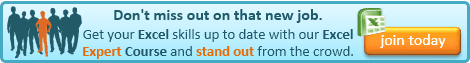## Tuesday, 24 September 2013

### Excel Tip: Case Sensitive VLOOKUP

When I get stuck on Excel, I usually find myself Googling the forums for an answer, as was the case last week, when I needed a case-sensitive VLOOKUP for a job I was doing for a client.

The Microsoft site suggests a solution that doesn't really work! It only checks that the answer found is the right case. This is no use if your data has the same text in different cases (as my client's did).

However, I did find an ingenious solution, which I thought I would share with you - as well as explain how it works.

I found my answer by DonkeyOte on this Excel Forum discussion.

"There are a few approaches - assume lookup values in A with values to be returned in B ... criteria is in C1 with result formula in D1:

D1: =INDEX(B1:B100,MATCH(TRUE,INDEX(EXACT(A1:A100,C1),0),0))"

If you are not interested in how it works, you can just use it as is and just replace as follows:

A1:A100 with the column (or row) range you want to lookup in
C1 with the lookup value
B1:B100 with the column (or row) range containing the results

(Use row ranges if you want to replicate an HLOOKUP)

If you want to know how it works, read on...

As you can see, this uses three separate functions - one of them twice.

I have covered using MATCH and INDEX together to create a VLOOKUP before.

Here is my introduction of these two functions from that post:

"INDEX is a function for returning a cell or range from within an array. At its simplest level this is done by referring to the cell by its row and column number (INDEX can do quite a bit more than this and also has another form which allows you to look at multiple ranges, however we only need to use its simple form here - I may do a post on some of its more advanced features at a later date). The simple form of INDEX is as follows:

=INDEX(range,row,column)

Column can be omitted and, if so, it is assumed to be 1 - unless range is just a single column in which case Excel will assume that the omitted argument is the row.

So for example:

=INDEX(A1:D5,2,3) returns the value in C2

MATCH finds the position of a value in a single row or column range. Its syntax is:

=MATCH(lookup value,range,match type)

match type is optional and has the following three possible values:

1 (or omitted)  - finds the position of the largest value that is less than or equal to lookup value and requires the range to be in ascending order (this works the same way as using TRUE for the 4th argument in a VLOOKUP).

-1  - finds the position of the smallest value that is greater than or equal to lookup value and requires the range to be in descending order.

0  - finds the position of the first value that is exactly equal to lookup value (this works the same way as using FALSE for the 4th argument of VLOOKUP). In this case, the range can be in any order."

DonkeyOte's formula uses the principles from my earlier post, but uses the EXACT funtion to deal with the case in a very clever way.

EXACT compares two values and returns TRUE if they are exactly the same (case as well), and FALSE if they are not. Our formula compares a range to our lookup value using EXACT.

EXACT(A1:A100,C1) will return a series of 100 TRUEs and FALSEs, the position of the first TRUE, being the position of the row that agrees to C1, our lookup value.

We can use INDEX to convert that to an array, as a column number of 0 will return the whole column as an array. So,

INDEX(EXACT(A1:A100,C1),0) returns the 100 TRUEs and FALSEs as an array

We can then use MATCH to determine the position of the first TRUE so,

MATCH(TRUE,INDEX(EXACT(A1:A100,C1),0),0) returns the position of the first TRUE

Finally, we use INDEX to return our result, being the contents of the same row in column B,

INDEX(B1:B100,MATCH(TRUE,INDEX(EXACT(A1:A100,C1),0),0))

Very clever, I thought!If you enjoyed this post, go to the top of the blog, where you can subscribe for regular updates and get your free report "The 5 Excel features that you NEED to know".

1.My brain hurts!
Jonathan

1.Mine did, that's why I suggested that the explanation was optional! :0)

2.without the explaination I failed to translate to German, so thanks for the hint and special thanks for the explanation! Just in case somebody is interested in: =INDEX(B1:B6;VERGLEICH(WAHR;INDEX(IDENTISCH(A1:A6;D1);0);0))
(with a little help of http://www.piuha.fi/excel-function-name-translation/index.php?page=english-german.html)

3.2.You are a star! I googled for an hour before I found this. I too found Microsoft answered the problem by saying you could only check whether it had returned the correct answer or not!!

1.3.4.5.6.